Gradient descent is an optimization algorithm used to minimize some function by iteratively moving in the direction of steepest descent as defined by the negative of the gradient. In machine learning, we use gradient descent to update the parameters of our model.

Learning rate
The size of these steps is called the learning rate. With a high learning rate we can cover more ground each step, but we risk overshooting the lowest point since the slope of the hill is constantly changing. With a very low learning rate, we can confidently move in the direction of the negative gradient since we are recalculating it so frequently. A low learning rate is more precise, but calculating the gradient is time-consuming, so it will take us a very long time to get to the bottom.

Cost function
A Loss Functions tells us “how good” our model is at making predictions for a given set of parameters. The cost function has its curve and its gradients. The slope of this curve tells us how to update our parameters to make the model more accurate.

Step-by-step
Now let’s run gradient descent using our new cost function. There are two parameters in our cost function that we can control: m (weight) and b (bias). Since we need to consider the impact each one has on the final prediction, we need to use partial derivatives. We calculate the partial derivatives of the cost function concerning each parameter and store the results in a gradient.

To solve for the gradient, we iterate through our data points using our new m and b values and compute the partial derivatives. This new gradient tells us the slope of our cost function at our current position (current parameter values) and the direction we should move to update our parameters. The size of our update is controlled by the learning rate.

Code

def update_weights(m, b, X, Y, learning_rate):    m_deriv = 0    b_deriv = 0    N = len(X)    for i in range(N):        # Calculate partial derivatives        # -2x(y - (mx + b))        m_deriv += -2*X[i] * (Y[i] - (m*X[i] + b))# -2(y - (mx + b))        b_deriv += -2*(Y[i] - (m*X[i] + b))# We subtract because the derivatives point in direction of steepest ascent    m -= (m_deriv / float(N)) * learning_rate    b -= (b_deriv / float(N)) * learning_ratereturn m, b

So we have our hypothesis function and we have a way of measuring how well it fits into the data. Now we need to estimate the parameters in the hypothesis function. That’s where gradient descent comes in.

Imagine that we graph our hypothesis function based on its fields θ0\theta_0θ0​ and θ1\theta_1θ1​ (actually we are graphing the cost function as a function of the parameter estimates). We are not graphing x and y itself, but the parameter range of our hypothesis function and the cost resulting from selecting a particular set of parameters.

We put θ0\theta_0θ0​ on the x-axis and θ1\theta_1θ1​ on the y-axis, with the cost function on the vertical z-axis. The points on our graph will be the result of the cost function using our hypothesis with those specific theta parameters. The graph below depicts such a setup.

We will know that we have succeeded when our cost function is at the very bottom of the pits in our graph, i.e. when its value is the minimum. The red arrows show the minimum points in the graph.

The way we do this is by taking the derivative (the tangential line to a function) of our cost function. The slope of the tangent is the derivative at that point and it will give us a direction to move towards. We make steps down the cost function in the direction with the steepest descent. The size of each step is determined by the parameter α, which is called the learning rate.

For example, the distance between each ‘star’ in the graph above represents a step determined by our parameter α. A smaller α would result in a smaller step and a larger α results in a larger step. The direction in which the step is taken is determined by the partial derivative of J(θ0,θ1)J(\theta_0,\theta_1)J(θ0​,θ1​). Depending on where one starts on the graph, one could end up at different points. The image above shows us two different starting points that end up in two different places.

repeat until convergence:

In this video we explored the scenario where we used one parameter theta 1 and plotted its cost function to implement gradient descent. Our formula for a single parameter was :

Repeat until convergence:

# Gradient Descent For Linear Regression

The point of all this is that if we start with a guess for our hypothesis and then repeatedly apply these gradient descent equations, our hypothesis will become more and more accurate.

So, this is simply gradient descent on the original cost function J. This method looks at every example in the entire training set on every step, and is called batch gradient descent. Note that, while gradient descent can be susceptible to local minima in general, the optimization problem we have posted here for linear regression has only 1 global, and no other local, optima; thus gradient descent always converges (assuming the learning rate α is not too large) to the global minimum. Indeed, J is a convex quadratic function. Here is an example of gradient descent as it is run to minimize a quadratic function.

The ellipses shown above are the contours of a quadratic function. Also shown is the trajectory took by gradient descent, which was initialized at (48,30). The x’s in the figure (joined by straight lines) mark the successive values of θ that gradient descent went through as it converged to its minimum.

## More from Deepak Belwal

Army lover, Responsible_person, Influencer, Sharing Defence Knowledge, Joining the Armed Forces is my dream, Enthusiast Person, Parakarmo Vijayate, Jai Hind

Love podcasts or audiobooks? Learn on the go with our new app.

## 3-Step Data-lite Machine Learning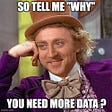## TensorFlow’s New Model MoveNet Explained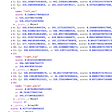## Predict Who Survived the Titanic Disaster## Paper review: “Learning to Represent Programs with Graphs”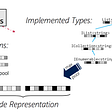## SLM Lab: New RL Research Benchmark & Software Framework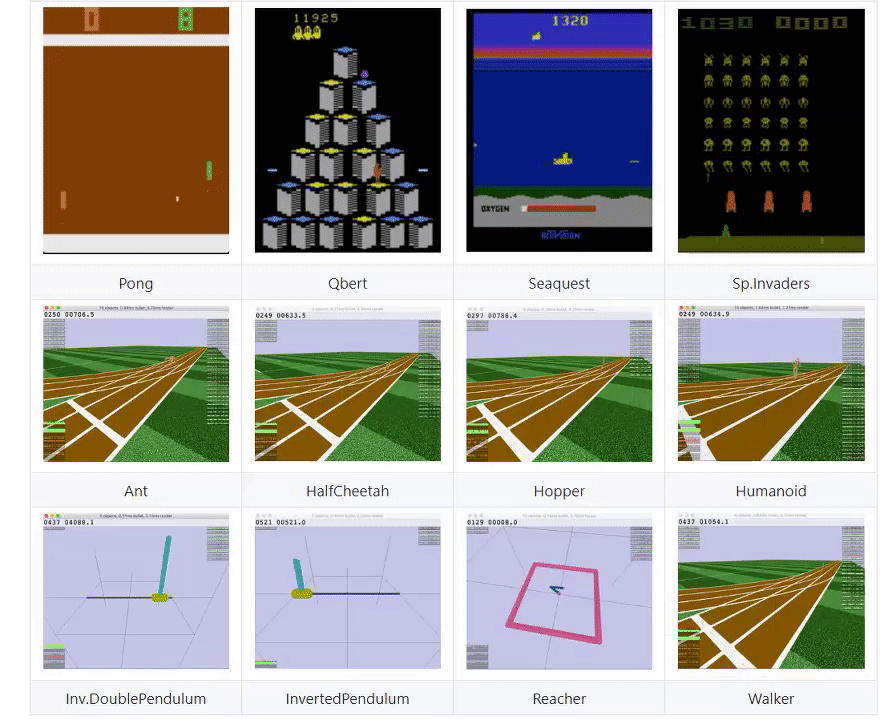## How to make a Pizza with Deep Learning## Face Aging with Conditional Generative Adversarial Networks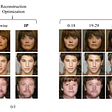## IBM Proposes Quantum-Enhanced Feature Space for ML## Deepak Belwal

Army lover, Responsible_person, Influencer, Sharing Defence Knowledge, Joining the Armed Forces is my dream, Enthusiast Person, Parakarmo Vijayate, Jai Hind

## Eigendecomposition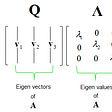## Emmy Noether, alphabetic portrait## Using CNN to predict regressive STD drug efficacy score## When post-modern neural networks feed from retro-pop culture